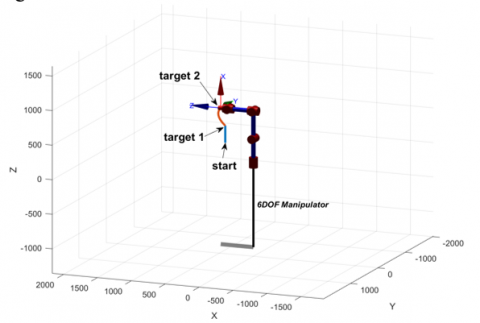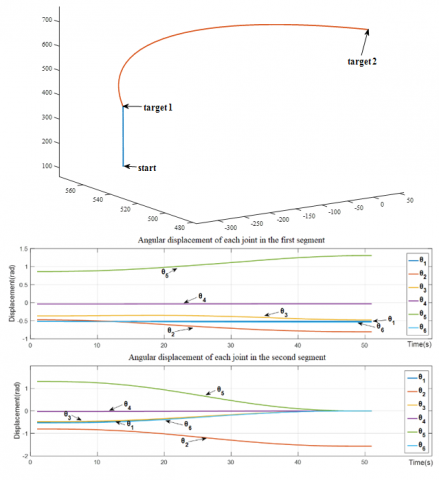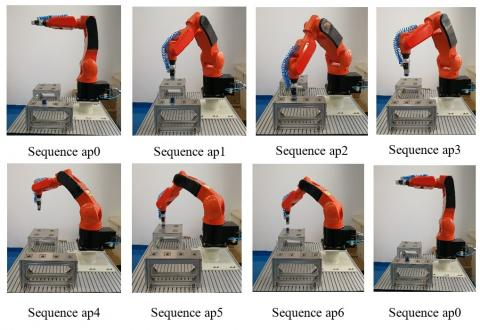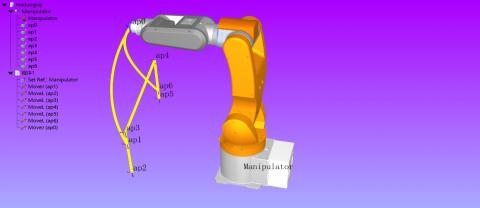# Kinematics Modelling and Experimental Analysis of a Six-Joint Manipulator

Kinematics Modelling and Experimental Analysis of a Six-Joint Manipulator
School of Mechanical Engineering, Baoji University of Arts and Sciences, Baoji 721007, China

Shaanxi Key Laboratory of Advanced Manufacturing and Evaluation of Robot Key Components, Baoji 721016, China

Corresponding Author Email:
mendezlee@bjwlxy.edu.cn
Page:
527-533
|
DOI:
https://doi.org/10.18280/jesa.520513
29 April 2019
|
Accepted:
7 August 2019
|
Published:
13 November 2019
| Citation

OPEN ACCESS

Abstract:

This paper establishes a kinematics model for a six degree-of-freedom (DOF) manipulator, and verifies its correctness through simulation and experiment. First, the model was set up through the D-H method. Then, the homogenous matrix transform was introduced to perform forward kinematics analysis and calculate the attitude of the end effector. Then, the manipulator position and trajectory were simulated on Robotics Toolbox for MATLAB and RoboDK. Finally, the simulated trajectory was verified through an experiment on stacking operation in the lab environment. The results show that the established kinematics model of the manipulator is correct, laying a solid theoretical basis for offline programming and calibration of manipulators.

Keywords:

Denavit and Hartenberg (D-H) parameters, manipulator, kinematics modelling, simulation

1. Introduction

With the introduction of Industry 4.0, industrial robots are increasingly widely used to satisfy the strong market demand. Many industrial robots adopt the 6 degree-of-freedom (DOF) manipulator . The kinematics modelling of the 6DOF manipulator provides the theoretical basis for trajectory planning [2-4], offline programming [5, 6] and calibration [7-9] of the manipulator.

The kinematics analysis of the manipulator is fundamental to controlling the motions and planning the trajectories of robot. The forward kinematics analysis is the most basic problem . The Denavit-Hartenberg (D-H) method, which is named after its creators, is a popular way for kinematics analysis [11, 12]. This method describes the spatial relationship between two adjacent links as a 4×4 homogenous transform matrix, and then establishes the kinematics equation of the manipulator . If applied to model the kinematics of the manipulator, the D-H method can convert the complex link motions into intuitive equations and matrix operations.

The MATLAB has been extensively adopted to simulate the kinematics of the manipulator [14, 15]. On the upside, the MATLAB greatly facilitates the kinematic simulation with its powerful computing ability. On the downside, this tool falls short in the simulation accuracy of manipulator motions. To solve the problem, an emerging tool, the 3D modelling software of robot, has attracted much attention from researchers. For example, the ADAMS is often used as a virtual prototype to simulate the manipulator trajectory [16, 17]. However, this software mainly targets dynamic simulation of mechanical systems, and face certain difficulties in kinematics simulation. Similarly, the RobotStudio [18, 19], an industrial robot-specific simulation software, has won high recognition for its easy operability. But this software only works for ABB industrial robots.

To make up for the defects of MATLAB simulation of manipulator kinematics, this paper establishes a kinematics model of a 6DOF manipulator and simulates the trajectory of the manipulator on RoboDK. Finally, an experiment on the manipulator was carried out in lab environment, aiming to verify the correctness of the establish kinematics model. The research lays the basis for offline programming and calibration of manipulators.

The remainder of this paper is organized as follows: Section 2 sets up a coordinate system for desktop 6DOF manipulator, and derives a kinematics model for the manipulator through the D-H method; Section 3 identifies the position and trajectory of the manipulator using Robotics Toolbox and RoboDK, respectively; Section 4 carries out a stacking operation with the manipulator in the lab environment to verify the established model; Section 5 wraps up this paper with several conclusions.

2. D-H Modelling

The manipulator with six rotary joints was placed on the operation platform, and a workstation (Figure 1) was established to design programs for the manipulator to grab and transfer materials.

## 1.jpgFigure 1. Workstation for manipulator experiment

To study the relative displacement between the links of the manipulator, a coordinate system was fixed rigidly onto each link according to the D-H method. The coordinate system fixed to the base is denoted as {0}, and that fixed to link i as {i}. In coordinate system {0}, axis Z0 of coordinate system {0} is collinear with the kinematics axis of joint 1, and points upward; axis X0 points to the reverse direction of axis Zi-1 along the common normal of axes Zi and Zi-1; axis Yi is formulated according to the rules of righthanded cartesian coordinate system. The coordinate systems of the links on the manipulator are displayed in Figure 2.

## 2.jpgFigure 2. The coordinate systems of the links on the manipulator

In Figure 2, θ1 and d1 are the rotation angle and distance from X0 to X1 about/along axis Z0, respectively; α1 and a1 are the rotation angle and distance from Z0 to Z1 about/along axis X0, respectively; …; θi and di are the rotation angle and distance from Xi-1 to Xi about/along axis Zi-1, respectively; αi and ai are the rotation angle and distance from Zi-1 to Z1 about/along axis Xi-1, respectively. The parameters ai, αi, di and θi are the D-H parameters of the links. They are used to describe each link and the relationship between the links. The D-H parameters of the 3kg 6DOF manipulator were obtained based on the coordinate systems of the links in Figure 2, and listed in Table 1 below.

Table 1. The D-H parameters of the manipulator

 i θi/(°) di/(mm) ai/(mm) αi/(°) Scope of work/° 1 θ1 (0) 344 0 -90 -165~165 2 θ2 (-90) 0 400 0 -180~0 3 θ3 (0) 0 0 -90 -180~50 4 θ4 (0) 366 0 90 -120~120 5 θ5 (0) 0 0 -90 -120~120 6 θ6 (0) 116 0 0 -360~360

According to the theory of homogenous transform, the link transform $^{i-1}_{i} T$ can be broken down into four sub-transforms:

(1) Making Xi-1 and Xi coplanar by rotating θi about axis Zi-1.

(2) Making Xi-1 and Xi colinear by translating di along axis Zi-1.

(3) Making the origins of two coordinate systems coincidental by translating ai along axis Xi-1.

(4) Making Xi-1 and Xi colinear by rotating $\alpha_{i}$ about axis Xi-1.

In this way, the transform matrix $^{i-1}_{i} T$ can be established as:

$^{i-1}_{i} T=\operatorname{Rot}\left(z, \theta_{i}\right) * \operatorname{Trans}\left(z, d_{i}\right) * \operatorname{Trans}\left(x, a_{i}\right)$$* \operatorname{Rot}\left(x, \alpha_{i}\right)$$=\left[\begin{array}{cccc}{c \theta_{i}}&{-s \theta_{i} * c \alpha_{i}} & {s \theta_{i} * s \alpha_{i}} & {a_{i} * c \theta_{i}} \\ {s \theta_{i}}&{ c \theta_{i} * c \alpha_{i}} & {-c \theta_{i} * s \alpha_{i}} & {a_{i} * s \theta_{i}} \\ {0} & {s \alpha_{i}} & {c \alpha_{i}} & {d_{i}} \\ {0} & {0} & {0} & {1}\end{array}\right]$  (1)

The transform matrix $^{i-1}_{i} T$ depends on the four parameters in Table 1. Here, a 6-dimensional set of joint vectors q=[q1 q2 q3 q4 q5 q6]T is introduced, with qi=θi being the vector of joint i. Because all six joints are rotary, the transform matrix $^{i-1}_{i} T$ is a function about q=[θ1 θ2 θ3 θ4 θ5 θ6]T.

The transform matrices of all links were multiplied to obtained the transform matrix of coordinate system {i} relative to coordinate system {0}:

$_{6}^{0} T=_{1}^{0} T *_{2}^{1} T *_{3}^{2} T *_{4}^{3} T *_{5}^{4} T *_{6}^{5} T$    (2)

The above transform matrix can also be described by four vectors {n, a, o, p}:

$_{6}^{0} T=[n, o, a, p]=\left[\begin{array}{cccc}{n_{x}} & {o_{x}} & {a_{x}} & {p_{x}} \\ {n_{y}} & {o_{y}} & {a_{y}} & {p_{y}} \\ {n_{z}} & {o_{z}} & {a_{z}} & {p_{z}} \\ {0} & {0} & {0} & {1}\end{array}\right]$   (3)

where, a is the proximity vector; o is the orientation vector; n is the normal vector; p is the coordinates of the origin of coordinate system {i} in coordinate system {0}.

Combining formulas (2) and (3), we have the kinematics equation of the 6DOF manipulator. Substituting the D-H parameters and joint vectors, we have:

nx=s6(c4s1+s4(c1s2s3-c1c2c3))+c6(c5(s1s4-c4(c1s2s3-c1c2c3))-s5(c1c2s3+c1c3s2))

ny=-s6(c1c4-s4(s1s2s3-c2c3s1))-c6(c5(c1s4+c4(s1s2s3-c2c3s1))+s5(c2s1s3+c3s1s2))

nz=s4s6(c2s3+c3s2)-c6(s5(c2c3-s2s3)+c4c5(c2s3+c3s2))

ox=c6(c4s1+s4(c1s2s3-c1c2c3))-s6(c5(s1s4-c4(c1s2s3-c1c2c3))-s5(c1c2s3+c1c3s2))

oy=s6(c5(c1s4+c4(s1s2s3-c2c3s1))+s5(c2s1s3+c3s1s2))-c6(c1c4-s4(s1s2s3-c2c3s1))

oz=s6(s5(c2c3-s2s3)+c4c5(c2s3+c3s2))+c6s4(c2s3+c3s2)

ax=-s5(s1s4-c4(c1s2s3-c1c2c3))-c5(c1c2s3+c1c3s2)

ay=s5(c1s4+c4(s1s2s3-c2c3s1))-c5(c2s1s3+c3s1s2)

az=c4s5(c2s3+c3s2)-c5(c2c3-s2s3)

px=400c1c2-116s5(s1s4-c4(c1s2s3-c1c2c3))-(116c5+366)(c1c2s3+c1c3s2)

py=400c2s1+116s5(c1s4+c4(s1s2s3-c2c3s1))-(116c5+366)(c2s1s3+c3s1s2)

pz=-(366+116c5)(c2c3-s2s3)-400s2+116c4s5(c2s3+c3s2)+344

where, ci=cosθi and si=sinθi.

The above equation set represents the relationship between the link transform matrix $_{6}^{0} T$ of the 6DOF manipulator and the set of joint vectors q and provides the basis for kinematics analysis of the manipulator. Solving the link transform matrix $_{6}^{0} T$ based on joint vectors θ1, θ2, θ3, θ4, θ5 and θ6 is called the forward kinematics analysis, while solving the joint vectors θ1, θ2, θ3, θ4, θ5 and θ6 based on the link transform matrix $_{6}^{0} T$ is called the inverse kinematics analysis.

3. Kinematics Simulations

Our simulations were carried out using Robotics Toolbox and RoboDK. Robotics Toolbox is a tool embed in MATLAB for modeling, trajectory planning and control of manipulators. RoboDK is a simulation software to create a virtual working scene of robot and simulate the actual applications. The software supports multiple brands of manipulators and self-developed manipulator models. RoboDK simulations can approximate the actual working conditions of manipulators. Note that the D-H parameters are required for manipulator simulation by both MATLAB and RoboDK. The simulation can be completed using the relevant functions and commands. Meanwhile, the kinematics model of the manipulator is written into the underlying program, and remains invisible to users.

3.1 MATLAB simulation of manipulator position

The forward kinematics simulation of the manipulator aims to analyze the attitude change of the end effector according to the link parameters and the variation of each joint . Here, the manipulator position was simulated with Robotics Toolbox based on the established kinematics model. The simulation was carried out in the following steps:

Step 1. Based on the D-H parameters, the links were created on Robotics Toolbox, forming the manipulator, and a graph of the manipulator was produced.

Step 2. The set of joint vectors q=[q1 q2 q3 q4 q5 q6]T was adjusted randomly on the drive interface of the manipulator. Five different sets of joint vectors were selected: q1, q2, q3, q4 and q5. Then, the corresponding attitudes (x, y, z, $\varphi, \theta, \psi$) of the end effector were recorded.

Step 3. The five randomly selected sets of joint vectors were imputed to MATLAB, and the link transform matrix and end effector attitude of each set were solved using the user-defined program for the kinematics equation.

Through the above steps, the drive interface of the manipulator was obtained as Figure 3. In the interface, the link configuration of the manipulator is displayed on the right, the attitude of the end effector (x, y, z, $\varphi, \theta, \psi$) is shown on the upper left, and the adjustment region of joint vectors q1, q2, q3, q4, q5 and q6 is provided on the lower left. Because all joints are rotary, the joint vector qi equals the joint angle θi. Depending on the inputs in the adjustment region, the manipulator moved to the corresponding attitude, and the attitude parameters were displayed on the upper left of the interface.

## 3.jpgFigure 3. The drive interface of Robotics Toolbox

The six joint vectors in Figure 3 were adjusted, creating five sets of joint vectors (Table 2). The five sets of joint vectors were substituted into the program in Appendix 1. The results were collected and compared with the attitudes solved by MATLAB (Table 3).

As shown in Table 3, when five sets of joint vectors were randomly selected, the end effector attitudes obtained by Robotics Toolbox based on the D-H parameters were the same with those obtained by the kinematics equation, which was established based on the D-H method and homogenous matrix transform. Thus, the established kinematics model agrees well with the underlying model of Robotics Toolbox.

Table 2. The five randomly selected sets of joint vectors (unit: °)

 q q1(θ1) q2(θ2) q3(θ3) q4(θ4) q5(θ5) q6(θ6) q1 -70 -20 -31.55 44.29 -17.45 26.0 q2 -46.38 -42.44 20.85 -28.39 34.03 -18.07 q3 8.39 -91.56 -129.44 104.37 -80.68 23.25 q4 32.31 -53.47 -6.83 7.51 -32.65 23.25 q5 159.25 -105.72 30.97 -110.56 104.69 -99.57

Table 3. Comparison between the end effector attitudes obtained by Robotics Toolbox and those obtained by MATLAB

 Group Compare x/mm y/mm z/mm $\varphi$ /° $\theta$ /° $\psi$ /° q1 Robotics Toolbox 238.703 -726.852 203.905 -83.376 115.157 -142.830 Program calculation 238.703 -726.852 203.905 -83.376 115.157 -142.830 q2 Robotics Toolbox 261.979 -319.654 163.202 -166.252 162.130 16.691 Program calculation 261.979 -319.654 163.202 -166.252 162.130 16.691 q3 Robotics Toolbox -223.123 -144.995 1052.89 -76.910 73.567 64.748 Program calculation -223.123 -144.995 1052.89 -76.910 73.567 64.748 q4 Robotics Toolbox 571.985 352.055 489.584 28.262 87.281 -150.224 Program calculation 571.985 352.055 489.584 28.262 87.281 -150.224 q5 Robotics Toolbox -174.794 178.572 678.525 59.520 66.768 -20.137 Program calculation -174.794 178.572 678.525 59.520 66.768 -20.137

3.2 MATLAB simulation of manipulator trajectory

The first two segments of manipulator trajectory were planned and simulated. The set of joint vectors for a random initial configuration was set to qs=[-29.76, -26.987, -20.962, -2.252, 49.351, -30.017]T (unit: °). Through calculation, the initial attitude ($\mathrm{x}_{S}, \mathrm{y}_{S}, \mathrm{z}_{S}, \varphi_{S}, \theta_{S}, \psi_{S}$) of the end effector could be obtained as (541.286, -313.477, 164.455, -157.958, 177.827, 20.296). The set of joint vectors for target configuration 1 was qt1=[-29.761, -46.084, -27.357, -1.773, 74.754, -31.01]T (unit: °), and the corresponding attitude ($\mathrm{x}_{t 1}, \mathrm{y}_{t 1}, \mathrm{z}_{t 1}, \varphi_{t 1}, \theta_{t 1}, \psi_{t 1}$) of the end effector was (541.383, -313.553, 411.914, -157.112, 177.848, 21.153); The set of joint vectors for target configuration 2 was qt2=[0, -90, 0, 0, 0, 0]T (unit: °), and the corresponding attitude ($\mathrm{x}_{t 2}, \mathrm{y}_{t 2}, \mathrm{z}_{t 2}, \varphi_{t 2}, \theta_{t 2}, \Psi_{t 2}$) of the end effector was (482, 0, 744, 0, 90, -180). The relevant functions of Robotics Toolbox were adopted to plan the trajectory for the manipulator. The planned results are shown in Figure 4. The trajectory of the end effector and the angular displacement curve of each joint are presented in Figure 5.

## 4.pngFigure 4. The manipulator trajectory simulated by MATLAB

## 5.pngFigure 5. The trajectory of the end effector and the angular displacement curve of each joint

As shown in Figures 4 and 5, the first segment was a linear trajectory and the second segment, a curved trajectory; the manipulator ended up at the original position qt2=[0, -90, 0, 0, 0, 0]T (unit: °); all joints moved continuously and smoothly, the manipulator movement was stable, and the two segments were connected smoothly.

3.3 RoboDK simulation of manipulator trajectory

MATLAB integrates many basic algorithms of the manipulator. This greatly facilitates the research and simulation of traditional joint manipulator and mobile manipulator. However, the MATLAB simulation cannot reflect the collisions between links, for it overlooks the mechanical structure of the manipulator. To solve the defect, this paper simulates the first two segments of the trajectory in RoboDK again, using a real-scale 3D model of our manipulator.

The 3D structural map of the 6DOF manipulator were imported to RoboDK, and its D-K parameters were configured. Then, the sets of joint vectors at the initial configuration, target configuration 1 and target configuration 2 were demonstrated on the teach pendant screen, and named as q_start, q_target1 and q_target2, respectively. Through programming, the simulated manipulator trajectory is shown in Figure 6.

## 6.jpgFigure 6. The manipulator trajectory simulated by RoboDK

It can be seen that the manipulator trajectory simulated by RoboDK is the same with that simulated by MATLAB, and the q_start, q_target1 and q_target2 are consistent with the attitude computed in Subsection 4.2. In addition, all joints moved smoothly without any collision throughout the movement.

4. Experimental Analysis

This section plans a simple trajectory of the manipulator for stacking operation in the lab environment, and simulates the planned stacking trajectory on RoboDK. The simulation results were compared with the experimental data.

4.1 Trajectory planning in lab environment

The stacking operation aims to pick up a block from the right part of the workbench surface and place it on the left part of the workbench surface. To plan a suitable trajectory for the manipulator, it is necessary to identify the sequence of attitude nodes of the end effector, i.e. the sequence of ap0~ap6 (Figure 7).

According to the sequence of attitude nodes, the stacking operation was described as a series of motions and actions. The joint angles of the manipulator were read from the controller and recorded.

As shown in Table 4, the joint angles at the original position read on the controller were different from those in MATLAB. Through investigation, it is learned that the joint angle of the second link is -90° in MATLAB when the manipulator is at the original position. In actual operation, the controller will display 0° although the joint angle of the second link is inputted as -90°. Therefore, if the joint angle of the second link read from the controller is θ2, the corresponding value should be -θ2-90° in the MATLAB.

## 7.jpgFigure 7. The planned trajectory for the manipulator

Table 4. The process from pickup to placement

 Nodes Joint angles (θ1, θ2, θ3, θ4, θ5, θ6) /° Target ap0 (0,0,0,0,0,0) Original position ap1 (36.802, -35.09, 3.191, 0.453, 53.697, 35.855) Approaching the block ap2 (36.801, -52.229, 4.455, 0.649, 35.31, 35.599) Holding the block ap3 (36.801, -30.057, -0.032, 0.432, 61.996, 35.92) Lifting up the block ap4 (-29.761, -43.916, -27.357, -1.773, 74.754, -31.01) Approaching the destination ap5 (-29.76, -63.013, -20.962, -2.252, 49.315, -30.017) Placing the block ap6 (-29.76, -57.656, -20.128, -2.116, 53.9, -30.232) Raising the arm ap0 (0,0,0,0,0,0) Returning to the original position

4.2 RoboDK simulation

## 8.jpgFigure 8. The stacking trajectory simulated by RoboDK

The joint angles of the above sequence of attitude nodes were inputted to RoboDK. The stacking trajectory was simulated again (Figure 8), and the attitude was recorded at each node.

4.3 Comparative analysis

The attitudes at each node read from the controller were compared with those simulated by RoboDK (Table 5). The attitudes calculated by MATLAB based on the D-H method and homogenous matrix transform are also provided in Table 5. Each attitude is expressed as attitudes (x, y, z, $\varphi, \theta, \psi$); the coordinates (x, y, z) are given in mm; each posture ($\varphi, \theta, \psi$) is given in °.

As shown in Table 5, under the same inputs of joint angles, the attitudes simulated by RoboDK agree well with those computed by MATLAB. The attitudes read from the controller only had a slight difference from these two types of results. This means the manipulator motions are consistent with its kinematics model. Thus, the established kinematics model is correct, laying the basis for further research on manipulators.

Table 5. Comparison between attitudes

 Attitudes read from the controller Attitudes simulated by RoboDK Attitudes calculated by MATLAB ap0 (482.02, -0.01, 743.96,0, 90.01, -179.99) (482.0, 0, 744.0,0, 90.0, -180.0) (482.0, 0, 744.0,0, 90.0, -180.0) ap1 (410.52, 308.05, 328.67, -153.65, 177.99, 25.69) (410.52, 308.05, 328.63, -153.66, 177.99, 25.67) (410.52, 308.05, 328.63, -153.66, 177.99, 25.67) ap2 (410.45, 308.02, 167.21, -153.86, 177.97, 25.48) (410.46, 308.02, 167.27, -153.86, 177.97, 25.47) (410.46, 308.02, 167.27, -153.86, 177.97, 25.47) ap3 (410.42, 308.01, 391.14, -153.88, 177.94, 25.45) (410.42, 308.01, 391.15, -153.89, 177.94, 25.44) (410.42, 308.01, 391.15, -153.89, 177.94, 25.44) ap4 (541.38, -313.55, 411.91, -157.12, 17.85, 21.14) (541.38, -313.55, 411.91, -157.11, 17.85, 21.15) (541.38, -313.55, 411.91, -157.11, 17.85, 21.15) ap5 (541.28, -313.48, 164.46, -157.96, 177.83, 20.30) (541.29, -313.48, 164.46, -157.96, 177.83, 20.30) (541.29, -313.48, 164.46, -157.96, 177.83, 20.30) ap6 (541.15, -313.41, 219.14, -159.25, 177.79, 19.01) (541.15, -313.41, 219.14, -159.25, 177.79, 19.01) (541.15, -313.41, 219.14, -159.25, 177.79, 19.01)
5. Conclusions

This paper carries out modelling, simulation and experiment on a 3 kg removable 6DOF manipulator.

First, a kinematics model was set up for the 6DOF manipulator by the D-H method. A program was prepared in MATLAB according to the kinematics equation. Using this program, the transform matrix $_{6}^{0} T$ of coordinate system {i} relative to coordinate system {0} and the attitude of the end effector (x, y, z, $\varphi, \theta, \psi$) can be derived from the 6 joint angles.

Second, the manipulator position and trajectory were simulated on Robotics Toolbox, and verified through a simulation on RoboDK. The results show that the established kinematics model agrees with the underlying model of RoboDK, and the manipulator movement was smooth and free of collisions.

Third, a simple stacking operation was performed in lab environment, and a sequence of attitude nodes was set up for the operation. The attitudes at each node were read from the controller, simulated by RoboDK and calculated by MATLAB based on the kinematics equation. The comparison between these results demonstrates the correctness of the established kinematics model.

The above work confirms that the established kinematics model is correct, and can serve as a reliable theoretical model for offline programming for the manipulator. The research results lay the basis for developing calibration techniques for manipulators.

Acknowledgment

This paper is supported by Key Research and Development Plan of Shaanxi Province, China (Grant No.: 2019GY-091); General Innovative Research Projects for Graduate Students, Baoji University of Arts and Sciences (Grant No.: YJSCX18YB37); Key Innovative Research Projects for Graduate Students, Baoji University of Arts and Sciences (Grant No.: YJSCX18ZD05).

References

 Ranjbaran, F., Angeles, J., González-Palacios, M.A., Patel, R.V. (1995). The mechanical design of a seven-axes manipulator with kinematic isotropy. Journal of Intelligent and Robotic Systems, 14(1): 21-41. https://doi.org/10.1007/BF01254006

 Wei, K., Ren, B. (2018). A method on dynamic path planning for robotic manipulator autonomous obstacle avoidance based on an improved RRT algorithm. Sensors, 18(2): 571. https://doi.org/10.3390/s18020571

 Chen, X.F. (2018). Research on the trajectory planning algorithm of palletizing robots. Control Engineering of China, 25(5): 925-929. https://doi.org/10.14107/j.cnki. kzgc.14D4.0033

 Fang, J., Song, Y., Zhu, M.F., Zhu, D.Q., Liu, Y.B., Jiao, J. (2018). Time-optimal trajectory planning for palletizing robots. Control Engineering of China, 25(1): 93-99. https://doi.org/10.14107/j.cnki.kzgc.150879

 Gołda, G., Kampa, A. (2017). Manipulation and handling processes off-line programming and optimization with use of K-Roset. IOP Conference Series: Materials Science and Engineering, 227(1): 1-6. https://doi.org/10.1088/1757-899X/227/1/012050

 Ni, W.B., Yang, W.C. (2017). The research on a method of off-line motion planning for dual-arm robot. Manufacturing Technology & Machine Tool, (4): 25-28. https://doi.org/10.19287/j.cnki.1005-2402.2017.04.001

 Joubair, A., Bonev, I.A. (2015). Kinematic calibration of a six-axis serial robot using distance and sphere constraints. The International Journal of Advanced Manufacturing Technology, 77(1-4): 515-523. https://doi.org/10.1007/s00170-014-6448-5

 Wu, L., Ren, H. (2016). Finding the kinematic base frame of a robot by hand-eye calibration using 3D position data. IEEE Transactions on Automation Science and Engineering, 14(1): 314-324. https://doi.org/10.1109/TASE.2016.2517674

 Zhou, X., Huang, S.F., Zhu, Z.H. (2019). TCP calibration model research and algorithm improvement of six joint industrial robot. Journal of Mechanical Engineering, 55(11): 186-196. https://doi.org/10.3901/JME.2019.11.186

 Liu, J.J., Peng, J.Q., Liu, X.H. (2015). Forward kinematics analysis and simulation for a series parallel hybrid 7-DOF humanoid mechanical arm based on MATLAB. Journal of Mechanical Transmission, 39(7): 59-62, 66. https://doi.org/10.16578/j.issn.1004.2539.2015.07.014

 Hartenberg, R.S., Denavit, J. (1955). A kinematic notation for lower pair mechanisms based on matrices. Journal of applied mechanics, 77(2): 215-221.

 Zhang, X., Zheng, Z.L., Qi, Y. (2016). Parameter identification and calibration of D-H model for 6-DOF serial robots. Robot, 38(3): 360-370. https://doi.org/10.13973/j.cnki.robot.2016.0360

 Shimizu, M., Kakuya, H., Yoon, W.K., Kitagaki, K., Kosuge, K. (2008). Analytical inverse kinematic computation for 7-DOF redundant manipulators with joint limits and its application to redundancy resolution. IEEE Transactions on Robotics, 24(5): 1131-1142. https://doi.org/10.1109/TRO.2008.2003266

 Huang, Y., Wang, J., Tham, D.M. (2017). Kinematics modelling and adams matlab/simulink co-simulation for automated aerobridge docking process. In 2017 3rd International Conference on Control, Automation and Robotics (ICCAR), 304-309. https://doi.org/10.1109/ICCAR.2017.7942708

 Li, X.H., Sun, Q., Zhang, L.G., Zhang, J. (2018). Research and simulation of the kinematics of dual-arm 6R service robot. Journal of Mechanical Transmission, 42(5): 129-134. https://doi.org/10.16578/j.issn.1004.2539.2018.05.026

 Wen, G., Xu, L., He, F., Zhang, X. (2009). Kinematics simulation to manipulator of welding robot based on ADAMS. In 2009 International Workshop on Intelligent Systems and Applications, 1-4. https://doi.org/10.1109/IWISA.2009.5072932

 Zhu, H.J. (2019). Kinematic analysis of 5-DOF welding robot based on screw theory. Machine Building & Automation, 48(1): 150-152. https://doi.org/10.19344/j.cnki.issn1671-5276.2019.01.039

 Zhang, G.Q., Spaak, A., Martinez, C., Lasko, D.T., Zhang, B., Fuhlbrigge, T.A. (2016). Robotic additive manufacturing process simulation-towards design and analysis with building parameter in consideration. In 2016 IEEE International Conference on Automation Science and Engineering (CASE), 609-613. https://doi.org/10.1109/COASE.2016.7743457

 Andrzej, B., Krzysztof, K., Dariusz, S., Magdalena, M., Jacek, N. (2017). Robot-operated quality control station based on the UTT method. Open Engineering, 7(1): 37-42. https://doi.org/10.1515/eng-2017-0008

 Fu, Y.Y., Ge, A.P. (2013). Kinematics simulation study based on MATLAB on industrial robot. Mechanical Engineering & Automation, (3): 40-42. https://doi.org/10.3969/j.issn.1672-6413.2013.03.018